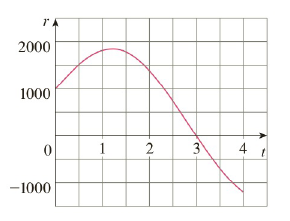Chapter 4.4, Problem 64E

Chapter
Section
Textbook Problem

Water flows into and out of a storage tank. A graph of the rate of change r(t) of the volume of water in the tank, in liters per day, is shown. If the amount of water in the tank at time t = 0 is 25, 000 L, use the Midpoint Rule to estimate the amount of water in the tank four days later.To determine

To estimate:

The amount of water in the tank four days later by using the Midpoint Rule.

Explanation

1) Concept:

Use the Net Change theorem and the Midpoint Rule.

2) Theorem:

The integral of a rate of change is the net change.

abF'(x)dx=Fb-F(a)

3) Given:

The amount of water in the tank at t=0 is 25,000 L.

4) Calculation:

Initially, 25,000 L water is present in the tank.

Therefore, by the Net Change theorem, the amount of water in the tank four days later is given by

=25000+04r(t)dt

Find 04r(t)dt by using the Midpoint Rule.

With n=4, a=0 and b=4 interval width is

t=b-an

Substitute values.

t=4-04

t=1

And the midpoints are

t1-=12t0+t1=120+1=121=0.5

t2-=12t1+t2=121+2=123=1.5

t3-=12t2+t3=122+3=125=2

Still sussing out bartleby?

Check out a sample textbook solution.

See a sample solution

The Solution to Your Study Problems

Bartleby provides explanations to thousands of textbook problems written by our experts, many with advanced degrees!

Get Started

Prove the statement using the , definition of a limit. limx2(x2+2x7)=1

Single Variable Calculus: Early Transcendentals, Volume I

In Exercises 58, evaluate the expression. 7. |2 6|

Applied Calculus for the Managerial, Life, and Social Sciences: A Brief Approach

In words, explain what is measured by SS, variance, and standard deviation.

Statistics for The Behavioral Sciences (MindTap Course List)